Next: Evolution of Magnetic Rotators Up: ``Ecology'' of Magnetic Rotators Previous: A Universal Diagram for

The Gravimagnetic Parameter

By expecting the expression for the stopping radiusĀ in the subcritical regime (), one can note that the magnetic dipole moment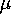and the accretion ratealways appear in the same combination,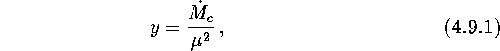as was first noticed by Davis and Pringle (1981). The parameter yĀ characterizes the ratio between the gravitational and magnetic ``properties'' of a star and will, therefore, be called the gravimagnetic parameter. Two magnetic rotators having quite different magnetic fields, subjected to different external conditions but with identical gravimagnetic parameters, have similar magnetospheres, as long as the accretion rate is quite low (). Otherwise, the flux of matter near the stopping radius no longer depends on the accretion rate at a large distance.

In fact, the number of independent parameters can be further reduced (see Osminkin and Prokhorov, 1995) by introducing the parameter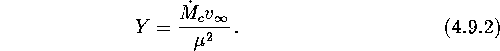Plotting the rotator's period p versus the Y-parameterĀ we can draw a somewhat less obvious but more general classification diagramĀ than the ``'' diagram discussed above. This permits us to show on a single plot the rotators with key parameters,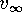andspanning a very wide range (Figure 9).

Ā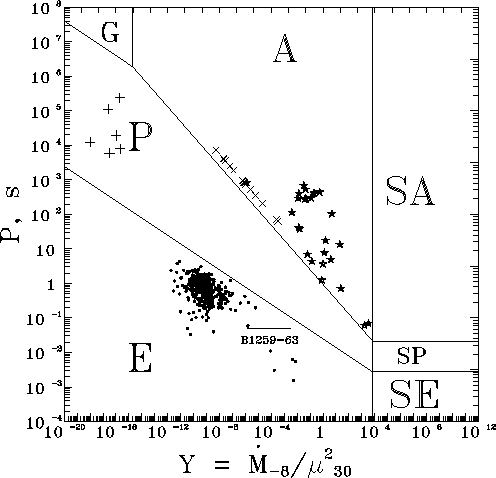Figure: The observed magnetic rotators on the universal period - gravimagnetic parameter ``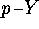'' diagram: ``+'', isolated WD (Lipunov and Nazin, 1992 );, intermediate polars;Ā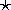, accreting NS;, radiopulsars. For isolated rotators, the accretion rate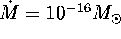Āyris assumed. The horizontal bar shows the orbital eccentricity-induced accretion rate change in binary pulsar PSR B1259-63.Ā

In the case of supercritical accretion,Ā another characteristic combination is found in all the expressions: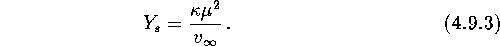Analoguous to the subcritical ``'' diagram, a supercritical ``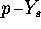'' diagram can be drawn.

Mike E. Prokhorov
Sat Feb 22 18:38:13 MSK 1997